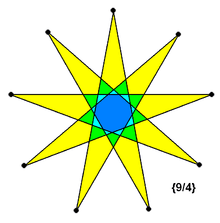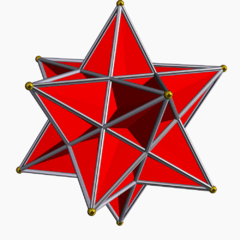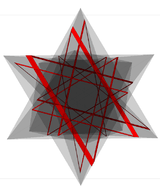# Density (polytope)The boundary of the regular enneagram {9/4} winds around its centre 4 times, so it has a density of 4.

In geometry, the density of a polytope represents the number of windings of a polytope, particularly a uniform or regular polytope, around its center. It can be visually determined by counting the minimum number of facet or face crossings of a ray from the center to infinity. The density is constant across any continuous interior region of a polytope that crosses no facets. For a non-self-intersecting (acoptic) polytope, the density is 1.

Tessellations with overlapping faces can similarly define density as the number of coverings of faces over any given point.

## Polygons

The density of a star polygon is the number of times that the polygonal boundary winds around its center; it is the winding number of the boundary around the central point.

For a regular star polygon {p/q}, the density is q.

It can be visually determined by counting the minimum number of edge crossings of a ray from the center to infinity.

## PolyhedraThe nonconvex great icosahedron, {3,5/2} has a density of 7 as demonstrated in this transparent and cross-sectional view on the right.

Arthur Cayley used density as a way to modify Euler's polyhedron formula (V E + F = 2) to work for the regular star polyhedra, where dv is the density of a vertex figure, df of a face and D of the polyhedron as a whole:

dv V E + df F = 2D 

For example, the great icosahedron, {3, 5/2}, has 20 triangular faces (df = 1), 30 edges and 12 pentagrammic vertex figures (dv = 2), giving

2·12 30 + 1·20 = 14 = 2D.

This implies a density of 7. The unmodified Euler's polyhedron formula fails for the small stellated dodecahedron {5/2, 5} and its dual great dodecahedron {5, 5/2}, for which VE + F = −6.

The regular star polyhedra exist in two dual pairs, with each figure having the same density as its dual: one pair (small stellated dodecahedron—great dodecahedron) has a density of 3, while the other (great stellated dodecahedron–great icosahedron) has a density of 7.

Hess further generalised the formula for star polyhedra with different kinds of face, some of which may fold backwards over others. The resulting value for density corresponds to the number of times the associated spherical polyhedron covers the sphere.

This allowed Coxeter et al. to determine the densities of the majority of the uniform polyhedra.

For hemipolyhedra, some of whose faces pass through the center, the density cannot be defined. Non-orientable polyhedra also do not have well-defined densities.

## Polychora

There are 10 regular star polychora or 4-polytopes (called the Schläfli–Hess polychora), which have densities between 4, 6, 20, 66, 76, and 191. They come in dual pairs, with the exception of the self-dual density-6 and density-66 figures.

## Notes

1. Coxeter, H. S. M; The Beauty of Geometry: Twelve Essays (1999), Dover Publications, LCCN 99-35678, ISBN 0-486-40919-8 (206–214, Density of regular honeycombs in hyperbolic space)
2. Cromwell, P.; Polyhedra, CUP hbk (1997), pbk. (1999). (Page 258)
3. Coxeter, 1954 (Section 6, Density and Table 7, Uniform polyhedra)

## References

• Coxeter, H. S. M.; Regular Polytopes, (3rd edition, 1973), Dover edition, ISBN 0-486-61480-8
• Coxeter, H. S. M.; Longuet-Higgins, M. S.; Miller, J. C. P. (1954), "Uniform polyhedra", Philosophical Transactions of the Royal Society of London. Series A. Mathematical and Physical Sciences, 246 (916): 401–450, doi:10.1098/rsta.1954.0003, ISSN 0080-4614, JSTOR 91532, MR 0062446
• Wenninger, Magnus J. (1979), "An introduction to the notion of polyhedral density", Spherical models, CUP Archive, pp. 132–134, ISBN 978-0-521-22279-2